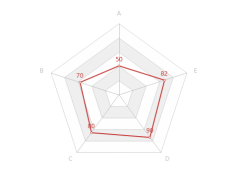{{ message }}

# e_radar some parameters don't work#98

Closed
opened this issue Jul 30, 2019 · 4 comments
Closed

# e_radar some parameters don't work#98

opened this issue Jul 30, 2019 · 4 comments

###hiffman commented Jul 30, 2019

 Hi John, when I want to change the radar layout, I find (center, radius )parameters don't work. Below is my code. ``````score_subset = data.frame( key = LETTERS[1:5], value = c(50,70,80,90,82) ) e_charts(score_subset, key) %>% e_radar(serie = value, max=100, legend = FALSE, center = c('20%', '45%'), radius=100) %>% e_labels(show = TRUE,position = "top") ``````The text was updated successfully, but these errors were encountered:

###JohnCoene commented Jul 30, 2019

 I'm afraid radar series do not have `center` parameter. What are you trying to accomplish?

###hiffman commented Jul 31, 2019

 I want to draw multiple radar, like this. In this example, I find center parameter can adjust position, radius parameter can adjust size.

###JohnCoene commented Aug 27, 2019

 OK. Coming back to this issue. I apologise, I was a bit hasty in saying it's not doable. There is indeed a `center` parameter but is passed to the `radar` indicator and not the radar `serie`. You can pass that `center` argument using `e_radar_opts`. Note that this function is actually already available in the CRAn version but is buggy so resintall from Github with ```install.packages("remotes") remotes::install_github("JohnCoene/echarts4r")``` I did try to work on better using the echarts4r grid but it was rather complicated in R and I realised that most of the types of charts (multiple charts that share interactions/legends) can actually be put together ver easily using arrange and connect so I forcused on the latter. The example below produces pretty much the same output as you want, ```df <- data.frame( x = LETTERS[1:5], y = runif(5, 1, 5), z = runif(5, 3, 7), w = runif(5, 2, 6) ) r1 <- df %>% e_charts(x, elementId = "radar1") %>% e_radar(y, max = 7) %>% e_radar_opts(center = c("50%", "35%")) r2 <- df %>% e_charts(x, elementId = "radar2") %>% e_radar(z, max = 7) r3 <- df %>% e_charts(x, elementId = "radar3") %>% e_radar(w, max = 7) %>% e_radar_opts(center = c("50%", "35%")) %>% e_connect(c("radar1", "radar2", "radar3")) # connect e_arrange(r1, r2, r3, cols = 3, rows = 1)``` I hope this helps.

###hiffman commented Oct 23, 2019

 It's a very good solution . Thank you !